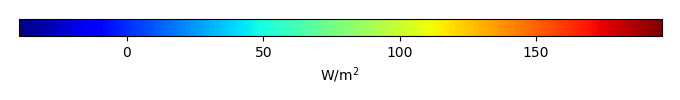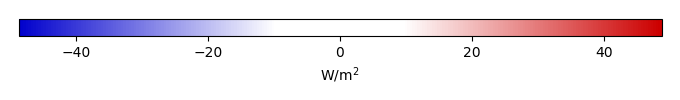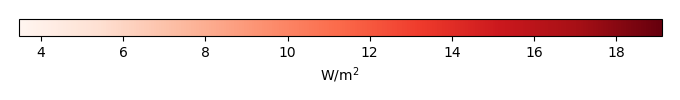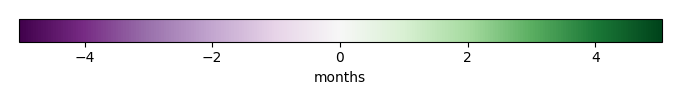# Mean State

Period Mean (original grids) [W/m2]
Model Period Mean (intersection) [W/m2]
Model Period Mean (complement) [W/m2]
Benchmark Period Mean (intersection) [W/m2]
Benchmark Period Mean (complement) [W/m2]
Bias [W/m2]
RMSE [W/m2]
Phase Shift [months]
Bias Score 
RMSE Score 
Seasonal Cycle Score 
Spatial Distribution Score 
Interannual Variability Score 
Overall Score 
Benchmark [-] 131.
CLM5PHSOFF [-] 115. 115. 130. 157. -14.2 23.1 1.40 0.504 0.418 0.815 0.994 0.714 0.644
CLM5PHSON [-] 116. 116. 130. 157. -13.7 22.6 1.40 0.513 0.420 0.812 0.990 0.694 0.642
Period Mean (original grids) [W/m2]
Model Period Mean (intersection) [W/m2]
Model Period Mean (complement) [W/m2]
Benchmark Period Mean (intersection) [W/m2]
Benchmark Period Mean (complement) [W/m2]
Bias [W/m2]
RMSE [W/m2]
Phase Shift [months]
Bias Score 
RMSE Score 
Seasonal Cycle Score 
Spatial Distribution Score 
Interannual Variability Score 
Overall Score 
Benchmark [-] 123.
CLM5PHSOFF [-] 96.1 95.6 122. 160. -26.2 31.5 1.41 0.353 0.499 0.790 0.923 0.695 0.627
CLM5PHSON [-] 97.4 96.9 122. 160. -24.8 30.3 1.43 0.374 0.505 0.789 0.941 0.650 0.627
Period Mean (original grids) [W/m2]
Model Period Mean (intersection) [W/m2]
Model Period Mean (complement) [W/m2]
Benchmark Period Mean (intersection) [W/m2]
Benchmark Period Mean (complement) [W/m2]
Bias [W/m2]
RMSE [W/m2]
Phase Shift [months]
Bias Score 
RMSE Score 
Seasonal Cycle Score 
Spatial Distribution Score 
Interannual Variability Score 
Overall Score 
Benchmark [-] 123.
CLM5PHSOFF [-] 104. 104. 121. 157. -17.7 25.7 1.10 0.571 0.598 0.850 0.938 0.731 0.714
CLM5PHSON [-] 105. 105. 121. 157. -15.0 25.1 1.49 0.616 0.570 0.774 0.955 0.683 0.695
Period Mean (original grids) [W/m2]
Model Period Mean (intersection) [W/m2]
Model Period Mean (complement) [W/m2]
Benchmark Period Mean (intersection) [W/m2]
Benchmark Period Mean (complement) [W/m2]
Bias [W/m2]
RMSE [W/m2]
Phase Shift [months]
Bias Score 
RMSE Score 
Seasonal Cycle Score 
Spatial Distribution Score 
Interannual Variability Score 
Overall Score 
Benchmark [-] 34.4
CLM5PHSOFF [-] 28.4 28.5 34.5 33.0 -5.96 21.4 0.284 0.842 0.724 0.981 0.831 0.732 0.806
CLM5PHSON [-] 32.9 33.0 34.5 33.0 -3.11 20.8 0.266 0.864 0.725 0.982 0.819 0.736 0.808
Period Mean (original grids) [W/m2]
Model Period Mean (intersection) [W/m2]
Model Period Mean (complement) [W/m2]
Benchmark Period Mean (intersection) [W/m2]
Benchmark Period Mean (complement) [W/m2]
Bias [W/m2]
RMSE [W/m2]
Phase Shift [months]
Bias Score 
RMSE Score 
Seasonal Cycle Score 
Spatial Distribution Score 
Interannual Variability Score 
Overall Score 
Benchmark [-] 147.
CLM5PHSOFF [-] 133. 132. 145. 156. -12.6 21.7 1.65 0.483 0.431 0.739 0.987 0.692 0.627
CLM5PHSON [-] 128. 127. 145. 156. -13.0 21.7 1.63 0.478 0.433 0.743 0.986 0.685 0.626
Period Mean (original grids) [W/m2]
Model Period Mean (intersection) [W/m2]
Model Period Mean (complement) [W/m2]
Benchmark Period Mean (intersection) [W/m2]
Benchmark Period Mean (complement) [W/m2]
Bias [W/m2]
RMSE [W/m2]
Phase Shift [months]
Bias Score 
RMSE Score 
Seasonal Cycle Score 
Spatial Distribution Score 
Interannual Variability Score 
Overall Score 
Benchmark [-] 53.7
CLM5PHSOFF [-] 42.8 42.7 53.5 64.9 -10.8 19.7 0.208 0.819 0.763 0.986 0.935 0.723 0.832
CLM5PHSON [-] 48.1 48.1 53.5 64.9 -5.14 17.0 0.214 0.875 0.774 0.986 0.903 0.721 0.839
Period Mean (original grids) [W/m2]
Model Period Mean (intersection) [W/m2]
Model Period Mean (complement) [W/m2]
Benchmark Period Mean (intersection) [W/m2]
Benchmark Period Mean (complement) [W/m2]
Bias [W/m2]
RMSE [W/m2]
Phase Shift [months]
Bias Score 
RMSE Score 
Seasonal Cycle Score 
Spatial Distribution Score 
Interannual Variability Score 
Overall Score 
Benchmark [-] 71.7
CLM5PHSOFF [-] 62.1 62.0 71.1 90.6 -9.09 19.7 0.442 0.793 0.717 0.963 0.994 0.696 0.813
CLM5PHSON [-] 62.6 62.6 71.1 90.6 -7.26 19.0 0.429 0.810 0.717 0.964 0.994 0.706 0.818
Period Mean (original grids) [W/m2]
Model Period Mean (intersection) [W/m2]
Model Period Mean (complement) [W/m2]
Benchmark Period Mean (intersection) [W/m2]
Benchmark Period Mean (complement) [W/m2]
Bias [W/m2]
RMSE [W/m2]
Phase Shift [months]
Bias Score 
RMSE Score 
Seasonal Cycle Score 
Spatial Distribution Score 
Interannual Variability Score 
Overall Score 
Benchmark [-] 120.
CLM5PHSOFF [-] 97.2 96.5 118. 153. -21.4 28.8 0.658 0.506 0.556 0.925 0.992 0.661 0.699
CLM5PHSON [-] 95.0 94.6 118. 153. -18.8 27.5 0.690 0.545 0.541 0.922 0.994 0.647 0.698
Period Mean (original grids) [W/m2]
Model Period Mean (intersection) [W/m2]
Model Period Mean (complement) [W/m2]
Benchmark Period Mean (intersection) [W/m2]
Benchmark Period Mean (complement) [W/m2]
Bias [W/m2]
RMSE [W/m2]
Phase Shift [months]
Bias Score 
RMSE Score 
Seasonal Cycle Score 
Spatial Distribution Score 
Interannual Variability Score 
Overall Score 
Benchmark [-] 30.5
CLM5PHSOFF [-] 21.8 22.2 31.0 19.5 -8.80 19.8 0.202 0.844 0.736 0.987 0.979 0.743 0.837
CLM5PHSON [-] 24.2 24.5 31.0 19.5 -7.63 19.3 0.205 0.860 0.736 0.986 0.981 0.754 0.842
Period Mean (original grids) [W/m2]
Model Period Mean (intersection) [W/m2]
Model Period Mean (complement) [W/m2]
Benchmark Period Mean (intersection) [W/m2]
Benchmark Period Mean (complement) [W/m2]
Bias [W/m2]
RMSE [W/m2]
Phase Shift [months]
Bias Score 
RMSE Score 
Seasonal Cycle Score 
Spatial Distribution Score 
Interannual Variability Score 
Overall Score 
Benchmark [-] 109.
CLM5PHSOFF [-] 94.0 93.9 108. 131. -13.8 20.9 0.505 0.728 0.729 0.962 0.945 0.796 0.815
CLM5PHSON [-] 93.6 93.6 108. 131. -12.2 20.1 0.514 0.751 0.727 0.960 0.956 0.769 0.815
Period Mean (original grids) [W/m2]
Model Period Mean (intersection) [W/m2]
Model Period Mean (complement) [W/m2]
Benchmark Period Mean (intersection) [W/m2]
Benchmark Period Mean (complement) [W/m2]
Bias [W/m2]
RMSE [W/m2]
Phase Shift [months]
Bias Score 
RMSE Score 
Seasonal Cycle Score 
Spatial Distribution Score 
Interannual Variability Score 
Overall Score 
Benchmark [-] 65.7
CLM5PHSOFF [-] 54.8 54.7 63.8 85.4 -9.07 18.1 0.215 0.851 0.779 0.986 0.986 0.675 0.843
CLM5PHSON [-] 54.7 54.7 63.8 85.4 -6.96 17.5 0.197 0.873 0.775 0.987 0.991 0.682 0.847
Period Mean (original grids) [W/m2]
Model Period Mean (intersection) [W/m2]
Model Period Mean (complement) [W/m2]
Benchmark Period Mean (intersection) [W/m2]
Benchmark Period Mean (complement) [W/m2]
Bias [W/m2]
RMSE [W/m2]
Phase Shift [months]
Bias Score 
RMSE Score 
Seasonal Cycle Score 
Spatial Distribution Score 
Interannual Variability Score 
Overall Score 
Benchmark [-] 109.
CLM5PHSOFF [-] 65.0 65.0 80.5 123. -15.5 23.9 0.654 0.620 0.615 0.924 0.979 0.693 0.741
CLM5PHSON [-] 65.1 65.1 80.5 123. -13.7 23.0 0.692 0.642 0.614 0.917 0.978 0.684 0.742
Period Mean (original grids) [W/m2]
Model Period Mean (intersection) [W/m2]
Model Period Mean (complement) [W/m2]
Benchmark Period Mean (intersection) [W/m2]
Benchmark Period Mean (complement) [W/m2]
Bias [W/m2]
RMSE [W/m2]
Phase Shift [months]
Bias Score 
RMSE Score 
Seasonal Cycle Score 
Spatial Distribution Score 
Interannual Variability Score 
Overall Score 
Benchmark [-] 76.6
CLM5PHSOFF [-] 55.2 55.1 73.9 144. -18.8 25.0 0.885 0.547 0.619 0.901 0.930 0.702 0.720
CLM5PHSON [-] 53.6 53.6 73.9 144. -18.0 24.8 0.888 0.556 0.615 0.901 0.940 0.708 0.723
Period Mean (original grids) [W/m2]
Model Period Mean (intersection) [W/m2]
Model Period Mean (complement) [W/m2]
Benchmark Period Mean (intersection) [W/m2]
Benchmark Period Mean (complement) [W/m2]
Bias [W/m2]
RMSE [W/m2]
Phase Shift [months]
Bias Score 
RMSE Score 
Seasonal Cycle Score 
Spatial Distribution Score 
Interannual Variability Score 
Overall Score 
Benchmark [-] 36.2
CLM5PHSOFF [-] 24.1 23.9 36.2 36.3 -12.3 22.1 0.0876 0.801 0.726 0.994 0.946 0.765 0.826
CLM5PHSON [-] 27.4 27.3 36.2 36.3 -10.9 21.5 0.0841 0.819 0.726 0.994 0.929 0.770 0.827
Period Mean (original grids) [W/m2]
Model Period Mean (intersection) [W/m2]
Model Period Mean (complement) [W/m2]
Benchmark Period Mean (intersection) [W/m2]
Benchmark Period Mean (complement) [W/m2]
Bias [W/m2]
RMSE [W/m2]
Phase Shift [months]
Bias Score 
RMSE Score 
Seasonal Cycle Score 
Spatial Distribution Score 
Interannual Variability Score 
Overall Score 
Benchmark [-] 138.
CLM5PHSOFF [-] 110. 110. 133. 161. -22.3 29.0 1.06 0.529 0.618 0.872 0.990 0.717 0.724
CLM5PHSON [-] 107. 107. 133. 161. -20.2 27.5 1.29 0.558 0.616 0.830 0.989 0.695 0.717
Period Mean (original grids) [W/m2]
Model Period Mean (intersection) [W/m2]
Model Period Mean (complement) [W/m2]
Benchmark Period Mean (intersection) [W/m2]
Benchmark Period Mean (complement) [W/m2]
Bias [W/m2]
RMSE [W/m2]
Phase Shift [months]
Bias Score 
RMSE Score 
Seasonal Cycle Score 
Spatial Distribution Score 
Interannual Variability Score 
Overall Score 
Benchmark [-] 98.6
CLM5PHSOFF [-] 80.1 79.9 96.9 135. -16.9 22.4 0.478 0.732 0.758 0.964 0.954 0.732 0.816
CLM5PHSON [-] 81.8 81.9 96.9 135. -13.3 19.7 0.468 0.783 0.765 0.965 0.928 0.729 0.822
Period Mean (original grids) [W/m2]
Model Period Mean (intersection) [W/m2]
Model Period Mean (complement) [W/m2]
Benchmark Period Mean (intersection) [W/m2]
Benchmark Period Mean (complement) [W/m2]
Bias [W/m2]
RMSE [W/m2]
Phase Shift [months]
Bias Score 
RMSE Score 
Seasonal Cycle Score 
Spatial Distribution Score 
Interannual Variability Score 
Overall Score 
Benchmark [-] 63.9
CLM5PHSOFF [-] 53.2 53.4 63.6 72.9 -10.3 18.5 0.149 0.834 0.782 0.990 0.991 0.722 0.850
CLM5PHSON [-] 58.2 58.3 63.6 72.9 -5.21 15.3 0.153 0.895 0.794 0.990 0.990 0.707 0.862
Period Mean (original grids) [W/m2]
Model Period Mean (intersection) [W/m2]
Model Period Mean (complement) [W/m2]
Benchmark Period Mean (intersection) [W/m2]
Benchmark Period Mean (complement) [W/m2]
Bias [W/m2]
RMSE [W/m2]
Phase Shift [months]
Bias Score 
RMSE Score 
Seasonal Cycle Score 
Spatial Distribution Score 
Interannual Variability Score 
Overall Score 
Benchmark [-] 82.3
CLM5PHSOFF [-] 68.6 68.8 81.8 110. -13.1 20.1 0.526 0.780 0.765 0.955 0.944 0.738 0.824
CLM5PHSON [-] 71.4 71.6 81.8 110. -9.78 18.5 0.542 0.815 0.767 0.952 0.949 0.744 0.832
Period Mean (original grids) [W/m2]
Model Period Mean (intersection) [W/m2]
Model Period Mean (complement) [W/m2]
Benchmark Period Mean (intersection) [W/m2]
Benchmark Period Mean (complement) [W/m2]
Bias [W/m2]
RMSE [W/m2]
Phase Shift [months]
Bias Score 
RMSE Score 
Seasonal Cycle Score 
Spatial Distribution Score 
Interannual Variability Score 
Overall Score 
Benchmark [-] 139.
CLM5PHSOFF [-] 121. 121. 138. 170. -16.7 24.5 1.09 0.350 0.398 0.851 0.981 0.744 0.620
CLM5PHSON [-] 121. 122. 138. 170. -15.8 24.0 1.23 0.359 0.398 0.823 0.974 0.724 0.613
Period Mean (original grids) [W/m2]
Model Period Mean (intersection) [W/m2]
Model Period Mean (complement) [W/m2]
Benchmark Period Mean (intersection) [W/m2]
Benchmark Period Mean (complement) [W/m2]
Bias [W/m2]
RMSE [W/m2]
Phase Shift [months]
Bias Score 
RMSE Score 
Seasonal Cycle Score 
Spatial Distribution Score 
Interannual Variability Score 
Overall Score 
Benchmark [-] 121.
CLM5PHSOFF [-] 105. 105. 120. 143. -15.5 24.0 0.868 0.626 0.614 0.906 0.845 0.737 0.724
CLM5PHSON [-] 107. 107. 120. 143. -12.8 22.6 0.892 0.665 0.612 0.897 0.818 0.703 0.718
Period Mean (original grids) [W/m2]
Model Period Mean (intersection) [W/m2]
Model Period Mean (complement) [W/m2]
Benchmark Period Mean (intersection) [W/m2]
Benchmark Period Mean (complement) [W/m2]
Bias [W/m2]
RMSE [W/m2]
Phase Shift [months]
Bias Score 
RMSE Score 
Seasonal Cycle Score 
Spatial Distribution Score 
Interannual Variability Score 
Overall Score 
Benchmark [-] 98.1
CLM5PHSOFF [-] 88.4 87.6 95.8 118. -8.41 24.3 0.514 0.748 0.703 0.954 0.991 0.638 0.789
CLM5PHSON [-] 90.4 89.8 95.8 118. -7.08 23.4 0.560 0.761 0.706 0.947 0.991 0.642 0.792

# Temporally integrated period mean

BENCHMARK MEANMODEL MEANBIASBIAS SCORERMSERMSE SCOREBENCHMARK INTERANNUAL VARIABILITYMODEL INTERANNUAL VARIABILITYINTERANNUAL VARIABILITY SCOREBENCHMARK MAX MONTHMODEL MAX MONTHDIFFERENCE IN MAX MONTHSEASONAL CYCLE SCORESPATIAL TAYLOR DIAGRAMMODEL COLORS# Spatially integrated regional mean

MODEL COLORSREGIONAL MEANANNUAL CYCLEMONTHLY ANOMALYANNUAL CYCLE# All Models

BenchmarkCLM5PHSOFFCLM5PHSON# Data Information

creation_date: Thu May 8 22:58:12 PDT 2014

Conventions: Please contact Prof. James Randerson (Email: jranders@uci.edu) or Dr. Mingquan Mu (mmu@uci.edu) for any question

source_file: This product is generated from monthly 1 degree CERES EBAF Radiation observations

title: derived CERES EBAF Radiation

Approach: I simply read this variable (sfc_net_tot_all_mon: surface all-sky net radiation) from original netcdf data file. Meanwhile, the data were also binned from original 1.0x1.0 resolution to final 0.5x0.5 resolution.

Temporal resolution: monthly

General information: This product was derived from CERES EBAF radiation dataset.

Spatial resolution: 0.5x0.5 degree